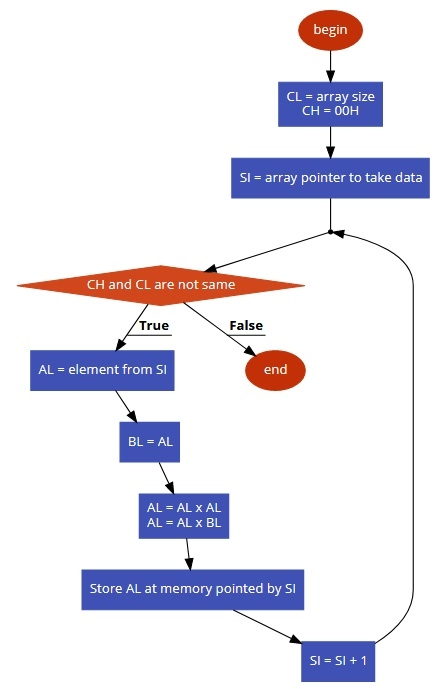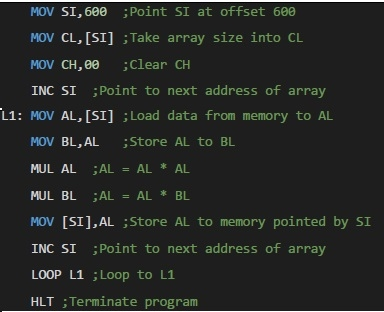# 8086 program to determine cubes of numbers in an array of n numbers

In this program we will see how to find the cubes of n numbers stored in an array.

## Problem Statement

Write 8086 Assembly language program to calculate cubes of each numbers stored in an array of size n. The array size is stored at location offset 600, and Numbers are stored at 601 onwards.

## Discussion

To solve this problem, we are taking the size of the array into the CL register, and make CH = 00H for counting. Now from each location take the number into accumulator, to make cube, we have to multiply it three times. so we are storing the number temporarily into another register, then multiply AL with AL, and then AL and the value again from that stored location. Thus the cube is generated. After that it is again stored into memory location.

Data

600
05
601
02
602
03
603
05
604
01
605
04

## Flow Diagram## ProgramData

601
08
602
1B
603
7D
604
01
605
40